# 一种基于PSS序列的OFDM系统低复杂高精度帧同步方法及其FPGA实现A Low Complex and High Precision Frame Synchronization Method for OFDM System Based on PSS Sequence and Its FPGA Implementation

• 全文下载: PDF(7222KB)    PP.45-56   DOI: 10.12677/HJWC.2018.82005
• 下载量: 421  浏览量: 1,438

Wireless frame synchronization is one of the key technologies in OFDM system. Only through frame synchronization between the base station and the user and the starting point of the wireless frame is found, communication signal can be successfully processed. Synchronization precision directly affects the performance of signal processing in the system. At the same time, how to reduce the complexity of the algorithm and reduce the consumption of hardware resources is also a key problem that must be considered in the algorithm design. In view of the above problems, a low complex and high precision frame synchronization algorithm is proposed in this paper. On the basis of traditional wireless frame synchronization, the algorithm only adds a plural multiplier, two accumulators and related logic modules. It can implement low complex and high precision wireless frame synchronization based on PSS sequence. Meanwhile, this paper implements the algorithm in hardware using LabVIEW FPGA language and the algorithm is deployed in the actual MIMO-OFDM system, operating stably and effectively.

1. 引言

2. 低复杂高精度帧同步算法

2.1. LTE中同步序列PSS

PSS序列是由长度为63点的ZC  序列(Zadoff-Chu)组成，本身为频域信号，具有自相关性极高互相关性极低的特点，通过IFFT (Inverse Fast Fourier Transformation)转换到时域，所得时域信号也具有同样的特点，适用于加入到无线帧的头部，可用于无线帧同步使用。

${a}_{q}=\mathrm{exp}\left[-j2\text{π}q\frac{n\left(n+1\right)/2+l*n}{{N}_{ZC}}\right]$ (1)

ZC序列有以下特性：

1) ZC序列具有恒定振幅，经过IFFT也是恒定振幅的。这限制了峰均值比，降低了对数据信号的干扰。

2) 任何长度的ZC序列具有极好的循环自相关性。

$Z{C}_{q}^{63}\left(n\right)=\mathrm{exp}\left[-j\frac{\text{π}qn\left(n+1\right)}{63}\right]$ (2)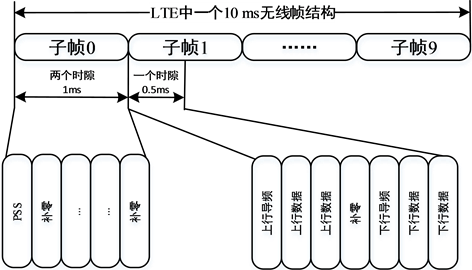Figure 1. Wireless frame formatFigure 2. Frequency domain signal of PSS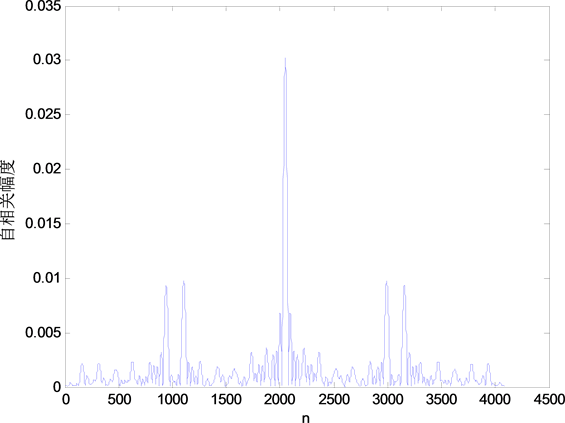Figure 3. PSS sequence autocorrelation

2.2. 粗同步算法

2.3. 高精度帧同步算法Figure 4. Block diagram of rough synchronization algorithm for OFDM systemFigure 5. The flow of high precision frame synchronization algorithm

3. 高精度无线帧同步的LabVIEW FPGA实现

LabVIEW FPGA是美国国家仪器公司推出的一款图形化界面FPGA开发语言   ，具有开发简单、可读性强等特点，被广泛应用于该公司的FPGA系列芯片的开发中，本论文采用该语言进行帧同步算法的实现。粗同步模块是高精度无线帧同步算法的必须步骤，首先简要介绍粗同步模块的实现    。Figure 6. 2048 point correlator input data range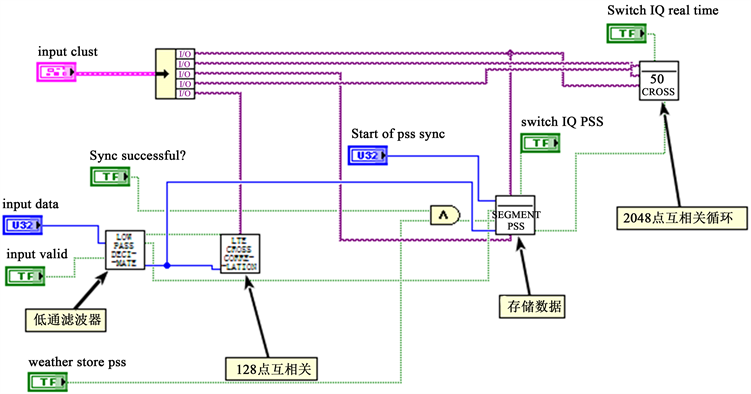Figure 7. Low pass sampling, cross correlation and other processes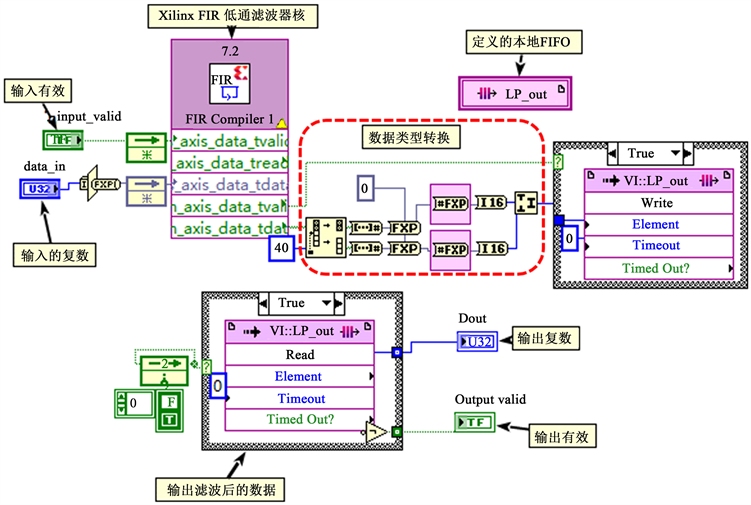Figure 8. Low pass filter module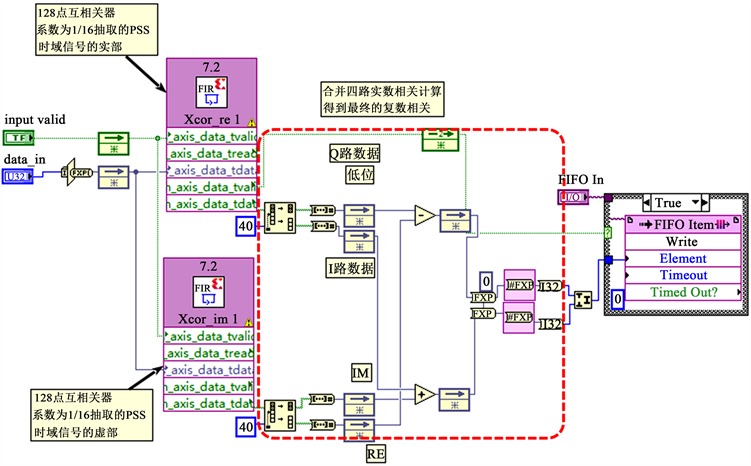Figure 9. 128 point cross-correlation

$\begin{array}{l}{C}_{n}=\underset{i=1}{\overset{128}{\sum }}\left(r{e}_{local,i}+i\ast i{m}_{local,i}\right){\left(r{e}_{time,n+i}+i\ast i{m}_{time,n+i}\right)}^{\ast }\\ \text{}=\underset{i=1}{\overset{128}{\sum }}\left[\left(r{e}_{local,i}\ast r{e}_{time,n+i}+i{m}_{local,i}\ast i{m}_{time,n+i}\right)+i\left(-r{e}_{local,i}\ast i{m}_{time,n+i}+i{m}_{local,i}\ast r{e}_{time,n+i}\right)\right]\\ \text{}=\underset{i=1}{\overset{128}{\sum }}r{e}_{local,i}\ast r{e}_{time,n+i}-i\ast \underset{i=1}{\overset{128}{\sum }}r{e}_{local,i}\ast i{m}_{time,n+i}+i\ast \underset{i=1}{\overset{128}{\sum }}i{m}_{local,i}\ast r{e}_{time,n+i}+\underset{i=1}{\overset{128}{\sum }}i{m}_{local,i}\ast i{m}_{time,n+i}\end{array}$ (3)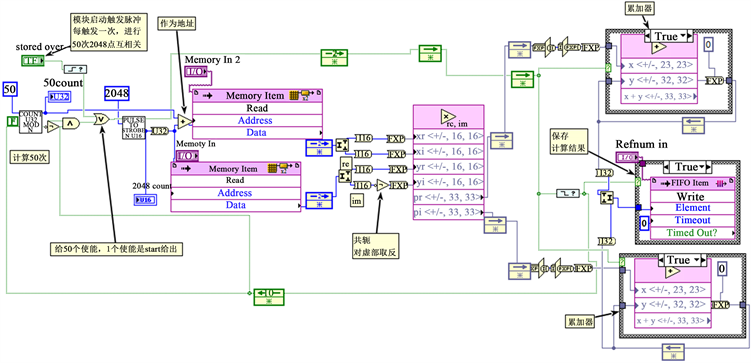Figure 10. The realization of 2048 cross-correlation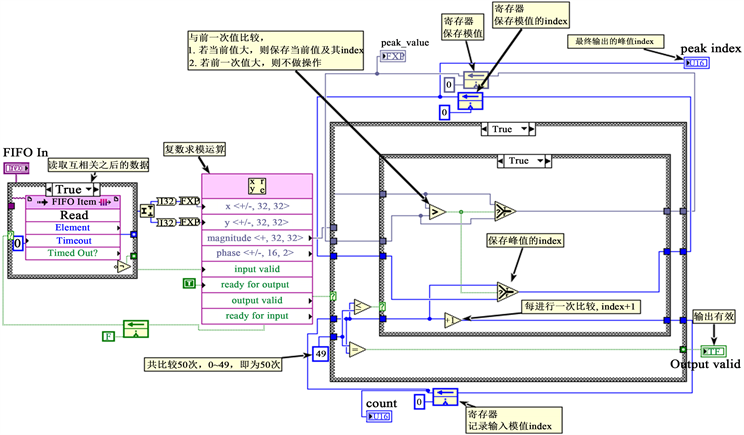Figure 11. 50 times peak search module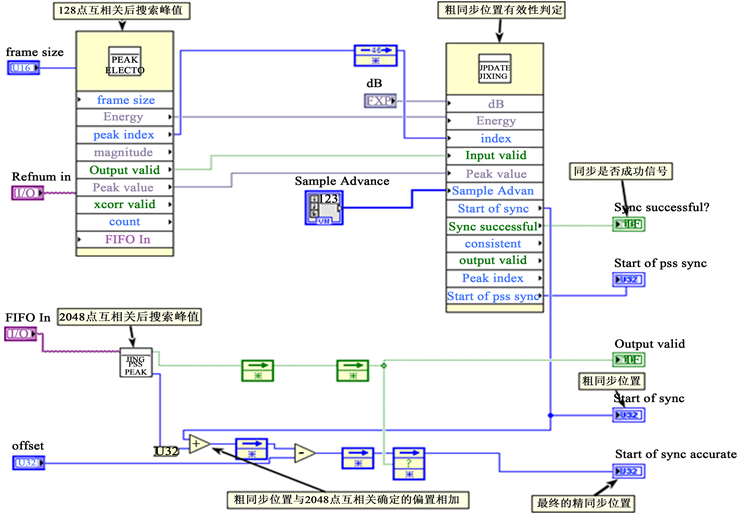Figure 12. Peak search and validity determination of synchronization results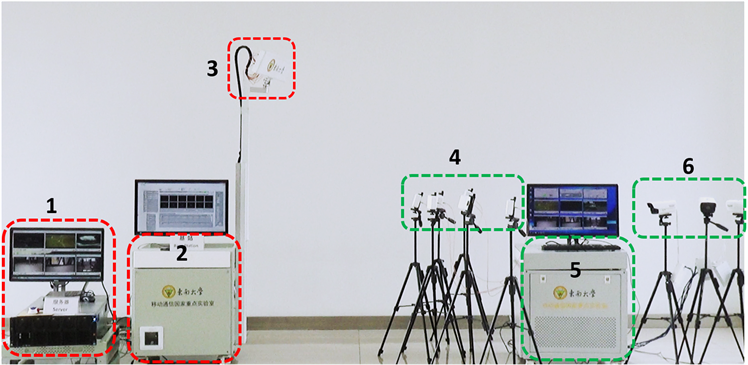Figure 13. The successful operation of this algorithm in the MIMO-OFDM system

4. 应用案例

5. 结论

  Yong Soo Cho, Jaekwon Kim, 等. MIMO-OFDM无线通信技术及MATLAB实现[M]. 北京: 电子工业出版社, 2014: 136-143.  赵邵刚, 李岳梦. LTE-Advanced宽带移动通信系统[M]. 北京: 人民邮电出版社, 2012.  Stefania Sesia, Issam Toufik, Matthew Baker. LTE/LTE-Advanced-UMTS长期演进理论与实践[M]. 北京:人民邮电出版社, 2012: 115-118.  Dahlman, E., Parkvall, S., Skold, J., et al. (2010) 3G Evolution: HSPA and LTE for Mobile Broadband. Academic Press, Cambridge.  阮奇桢. 我和LabVIEW: 一个NI工程师的十年编程经验[M]. 北京: 北京航空航天大学出版社, 2012.  严雨. LabVIEW入门与实战开发100例(第3版) [M]. 北京: 电子工业出版社, 2017.  Erik, L. (2014) 5G Massive MIMO Testbed: From Theory to Reality. http://www.ni.com/white-paper/52382/zhs/  刘东华. Xilinx系列FPGA芯片IP核详解[M]. 北京: 电子工业出版社, 2013: 127-156.  Yang, X., Huang, Z.C., Han, B., Zhang, S., Wen, C.K., Gao, F. and Jin, S. (2017) RaPro: A Novel 5G Rapid Prototyping System Architecture. IEEE Wireless Communications Letters, 6, 362-365. https://doi.org/10.1109/LWC.2017.2692780  National Instruments: NI USRP RIO. http://sine.ni.com/nips/cds/view/p/lang/zhs/nid/212991